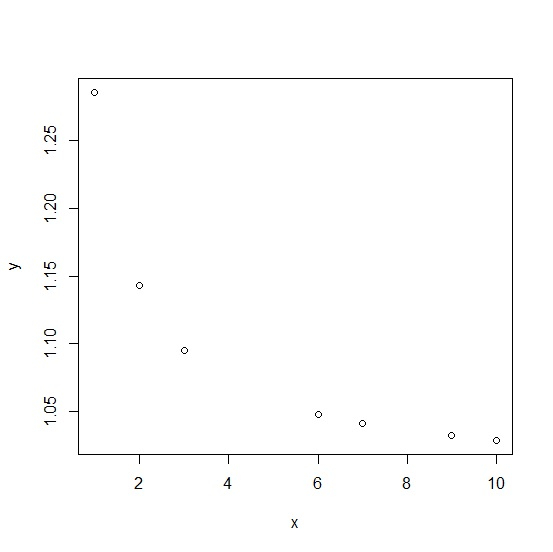# How to create a scatterplot between a variable and an equation in R?

To create a scatterplot between a variable and an equation in R, we can follow the below steps −

• First of all, create a vector
• Then, create the equation and store it in an object.
• After that, use plot function to create the plot between vector and equation object

## Create the vector

Let’s create a vector as shown below −

Live Demo

x<-sample(1:10,20,replace=TRUE)
x

On executing, the above script generates the below output(this output will vary on your system due to randomization) −

 6 9 1 7 3 6 6 2 7 6 1 2 6 7 7 10 3 3 1 1

## Create the equation

Let’s create an equation and save it in an object −

y<-1+(1/3.5)*(1/x)
y

On executing, the above script generates the below output(this output will vary on your system due to randomization) −

 1.047619 1.031746 1.285714 1.040816 1.095238 1.047619 1.047619 1.142857
 1.040816 1.047619 1.285714 1.142857 1.047619 1.040816 1.040816 1.028571
 1.095238 1.095238 1.285714 1.285714

## Create the plot between vector and equation

Using plot function to create the plot between vector x and equation y −

Live Demo

x<-sample(1:10,20,replace=TRUE)
y<-1+(1/3.5)*(1/x)
plot(x,y)

### Output